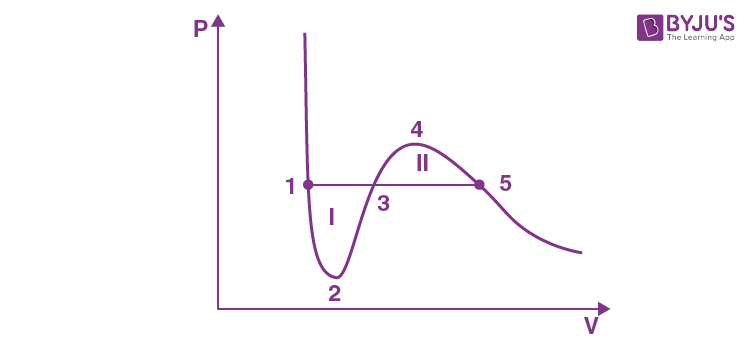# I.E. Irodov Solutions on Phase Transformations

Phase Transformations chapter 2.6 solved problems covered in I.E. Irodov textbook are available here. Students can get access to all sorts of problems based on each and every formula, equations and method. It is a complete capsule for the students who are curious to solve more and more problems related to the concept. Every problem is explicitly explained with a detailed solution which helps students to understand the concept in an easy way. All questions of I.E. Irodov book PART TWO. THERMODYNAMICS AND MOLECULAR PHYSICS - 2.6. Phase Transformations are available here. Students can easily get access to all varieties of questions based on Phase Transformations.

### I.E. Irodov Solutions on Phase Transformations

1. A saturated water vapour is contained in a cylindrical vessel under a weightless piston at a temperature t = 100°C. As a result of a slow introduction of the piston a small fraction of the vapour Δm = 0.70 g gets condensed. What amount of work was performed over the gas? The vapour is assumed to be ideal, the volume of the liquid is to be neglected.

Solution:

1. The condensation takes place at constant pressure and temperature and the work done is pΔV.

Where ΔV is the volume of the condensed vapour in the vapour phase. It is pΔV = (Δm/M)RT = 120.6J

Where M = 18 gm is the molecular weight of water.

2. A vessel of volume V = 6.0 l contains water together with its saturated vapour under a pressure of 40 atm and at a temperature of 250°C. The specific volume of the vapour is equal to V'c = 50 l/kg under these conditions. The total mass of the system water-vapour equals m = 5.0 kg. Find the mass and the volume of the vapour.

Solution:

1. The specific volume of water (the liquid) will be written as V'l since V'v >> V' l most of the weight is due to water. Thus if ml is mass of the liquid and mv that of the vapour then

m = ml + mv

V = ml V’l + mv V’v or V - m V’l = mv (V’v - V’l)

So, mv = [V-m V’l]/[ V’v - V’l] = 20 gm in the present case. Its volume is mvVv' = 1.01.

3. The saturated water vapour is enclosed in a cylinder under a piston and occupies a volume V0 = 5.0 l at the temperature t = 100°C. Find the mass of the liquid phase formed after the volume under the piston decreased isothermally to V = 1.6 l. The saturated vapour is assumed to be ideal.

Solution:

1. The volume of the condensed vapour was originally V0 - V at temperature T = 373 K. Its mass will be given by,

p(V0 - V) = (m/M) RT

on substituting the values, m = 2 gm where p is the atmospheric pressure.

4. A volume occupied by a saturated vapour is reduced isothermally n-fold. Find what fraction ri of the final volume is occupied by the liquid phase if the specific volumes of the saturated vapour and the liquid phase differ by N times (N > n). Solve the same problem under the condition that the final volume of the substance corresponds to the midpoint of a horizontal portion of the isothermal line in the diagram p, V.

Solution:

1. Let v'l be the specific volume of liquid.

Vv’ = N Vl’ is the Specific volume of vapour.

Let V = Original volume of the vapour.

Then,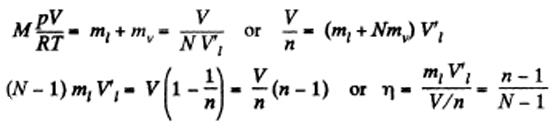In the case when the final volume of the substance corresponds to the midpoint of a horizontal portion of the isothermal line in the p y v diagram, the final volume must be (1+N)V'l/2 per unit mass of the substance.

Of this the volume of the liquid is V'1/2 per unit total mass of the substance.

Therefore, η = 1/(1+N)

5. An amount of water of mass m = 1.00 kg, boiling at standard atmospheric pressure, turns completely into saturated vapour. Assuming the saturated vapour to be an ideal gas find the increment of entropy and internal energy of the system.

Solution:

1. From the first law of thermodynamics

∆U + A = Q = mq

Where q is the specific latent heat of vaporization.

Now, A = p(Vv' - Vl')m = m(RT/M)

Therefore, ∆U = m(q – RT/M)

For water, this gives 2.08 x 106 joules (approx.)

6. Water of mass m = 20 g is enclosed in a thermally insulated cylinder at the temperature of 0°C under a weightless piston whose area is S = 410 cm2. The outside pressure is equal to standard atmospheric pressure. To what height will the piston rise when the water absorbs Q = 20.0 kJ of heat?

Solution:

1. Some of the heat used in heating water to the boiling temperature, T = 100°C = 373 K

The remaining heat = Q - mc Δ T (c = specific heat of water, ΔT - 100 K) is used to create vapour. If the piston rises to a height h then the volume of vapour will be sh (neglecting water). Its mass will be posh/RT x M and heat of vaporization will be poshMq/RT.

To this must be added the work done in creating the saturated vapour is approx. posh.

So, Q - mc Δ T = posh(1 + qM/RT)

or h = 20 cm [on substituting the values]

7. One gram of saturated water vapour is enclosed in a thermally insulated cylinder under a weightless piston. The outside pressure being standard, m = 1.0 g of water is introduced into the cylinder at a temperature t0 = 22°C. Neglecting the heat capacity of the cylinder and the friction of the piston against the cylinder's walls, find the work performed by the force of the atmospheric pressure during the lowering of the piston.

Solution:

1. A quantity mc(T-To)/q of saturated vapour must condense to heat the water to boiling point T = 373°K (Here c = specific heat of water, T0 = 295 K = initial water temperature).

The work done in lowering the piston = mc(T-To)/q x RT/M = 25 J

Since work done per unit mass of the condensed vapour is PV = RT/M.

8. If an additional pressure Δp of a saturated vapour over a convex spherical surface of a liquid is considerably less than the vapour pressure over a plane surface, then ∆p = (ρvl)2α/r where ρ0 and ρl are the densities of the vapour and the liquid, α is the surface tension, and r is the radius of curvature of the surface. Using this formula, find the diameter of water droplets at which the saturated vapour pressure exceeds the vapour pressure over the plane surface by η = 1.0% at a temperature t = 27°C. The vapour is assumed to be an ideal gas.

Solution:

1. Given, ∆p = (ρvl)2α/r = (ρvl)4α/d = [ηRT/M] ρv

Or d = 4 αM/ρ1RTη

For water a = 73 dynes/cm, M = 18gm, pl = gm/cc, T = 300 K, and with η = 0.01, we have

D = 0.2μm

9. Find the mass of all molecules leaving one square centimetre of water surface per second into a saturated water vapour above it at a temperature t = 100°C. It is assumed that η = 3.6% of all water vapour molecules falling on the water surface are retained in the liquid phase.

Solution:

1. In equilibrium the number of "liquid" molecules evaporating must equals the number of "vapour" molecules condensing.

Here p0 is atmospheric pressure and T = 373 K and M = molecular weight of water

By kinetic theory, this number is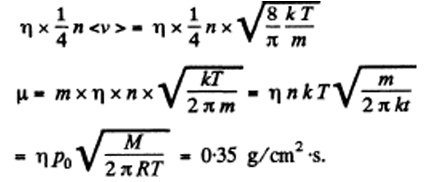10. Find the pressure of saturated tungsten vapour at a temperature T = 2000 K if a tungsten filament is known to lose a mass μ = 1.2-10-13 g/(s cm2) from a unit area per unit time when evaporating into high vacuum at this temperature.

Solution:

1. Here we must assume that i is also the rate at which the tungsten filament loses mass when in an atmosphere of its own vapour at this temperature and that η (of the previous problem) approx 1. Then,

p = μ √[2𝜋RT/M] = 0.9 n Pa

Where, p = pressure of the saturated vapour.

11. By what magnitude would the pressure exerted by water on the walls of the vessel have increased if the intermolecular attraction forces had vanished?

Solution:

1. From the Vander Waals equation: p = RT/(V-b) - a/V2

Where V = Volume of one gm mole of the substances.

For water V = 18 c.c. per mole = 1*8 x 10-2 litre per mole

a = 5.47 atoms . liter2/mole2

If molecular attraction vanished the equation will be, p' = RT/(V-b)

For the same specific volume.

∆p = a/V2 = 1.7 x 104 atoms (approx)

12. Find the internal pressure pi of a liquid if its density p and specific latent heat of vaporization q are known. The heat q is assumed to be equal to the work performed against the forces of the internal pressure, and the liquid obeys the Van der Waals equation. Calculate pi in water.

Solution:

1. The internal pressure being a/V2 the work done in condensation is

$\int_{v_l}^{V_s} \frac{a}{V^2} dV = \frac{a}{V_l}-\frac{a}{V_s} \approx \frac{a}{V_l}$

This by assumption is Mq, M being the molecular weight and Vl, Vg being the molar volumes of the liquid and gas.

Thus, pi = a/Vl2 = Mq/Vl = ρq, where ρ is the density of the liquid.

For water pi = 3-3 x 1013 atm

13. Demonstrate that Eqs. (2.6a) and (2.6b) are valid for a substance, obeying the Van der Waals equation, in critical state.

Instruction. Make use of the fact that the critical state corresponds to the point of inflection in the isothermal curve p(V).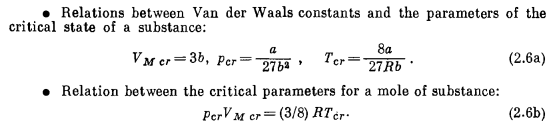Solution:

1. The Vander Waal's equation can be written as (for one mole)

p(V) = RT/(V-b) - a/V2

At the critical point vanish. Thus

$(\frac{\partial p}{\partial V})_T$ and $(\frac{\partial^2 p}{\partial V^2})_T$ vanih.

Therefore,

0 = RT/(V-b)2 + 2a/V3 or RT/(V-b)2 =- 2a/V3

0 = 2RT/(V-b)3 - 6a/V4 or RT/(V-b)3 = 3a/V4

Solving above equations,

V-b = 2/3 V, V = 3b = VMCr (approx.)

This is the critical molar volume. Putting this back, we get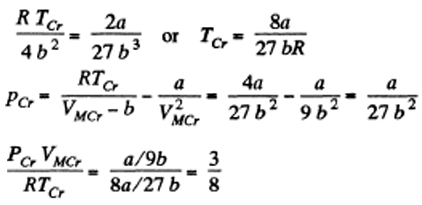14. Calculate the Van der Waals constants for carbon dioxide if its critical temperature Tcr = 304 K and critical pressure pcr = 73 atm.

Solution:

1.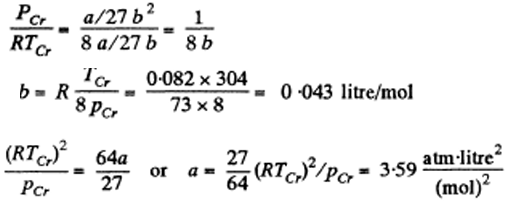15. Find the specific volume of benzene (C6H6) in critical state if its critical temperature Tcr = 562 K and critical pressure pcr = 47 atm.

Solution:

1. Specific volume is molar volume divided by molecular weight. Thus

V’cr = VMCr/M = 3RTCr/8MpCr = 4.71 cc/g

16. Write the Van der Waals equation via the reduced parameters π, v, and τ, having taken the corresponding critical values for the units of pressure, volume, and temperature. Using the equation obtained, find how many times the gas temperature exceeds its critical temperature if the gas pressure is 12 times as high as critical pressure, and the volume of gas is equal to half the critical volume.

Solution:

1.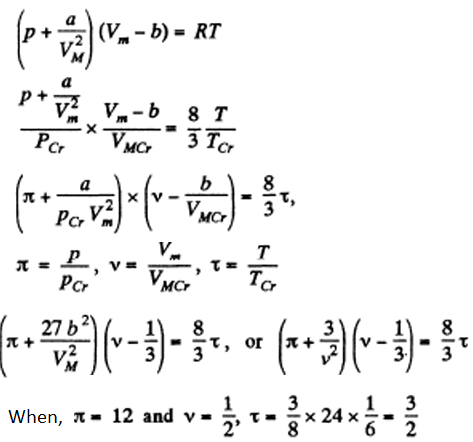17. Knowing the Van der Waals constants, find: (a) the maximum volume which water of mass m = 1.00 kg can occupy in liquid state; (b) the maximum pressure of the saturated water vapour.

Solution:

1. (a) The critical Volume VMCr is the maximum volume in the liquid phase and the minimum volume in the gaseous.

Therefore, Vmax = 5 liter (approx.)

(b) The critical pressure is the maximum possible pressure in the vapour phase in equilibrium with liquid phase.

Therefore, pmax = a/27b2 = 225 atm

18. Calculate the temperature and density of carbon dioxide in critical state, assuming the gas to be a Van der Waals one.

Solution:

1. TCr = (8/27)a/bR = (8/27) x 3.62/[.043x.082] = 304K (approx.)

ρCr = M/3b = 344/[3x43] gm/cc = 0.34 gm/cc

19. What fraction of the volume of a vessel must liquid ether occupy at room temperature in order to pass into critical state when critical temperature is reached? Ether has Tcr = 467 K, pcr =3 5.5 atm, M = 74 g/mol.

Solution:

1. The vessel is such that either vapour or liquid of mass m occupies it at critical point. Then its volume will be

VCr = (m/M)VMcr = (3/8)[RTcr/Pcr]m/M

The corresponding volume in liquid phase at room temperature is, V = m/ρ

where ρ = density of liquid ether at room temperature

η = V/Vcr = 8Mpcr/3RTcr ρ = 0.254 (approx.)

(using the given data and ρ = 720gm per litre)

20. Demonstrate that the straight line 1-5 corresponding to the isothermal-isobaric phase transition cuts the Van der Waals isotherm so that areas I and II are equal.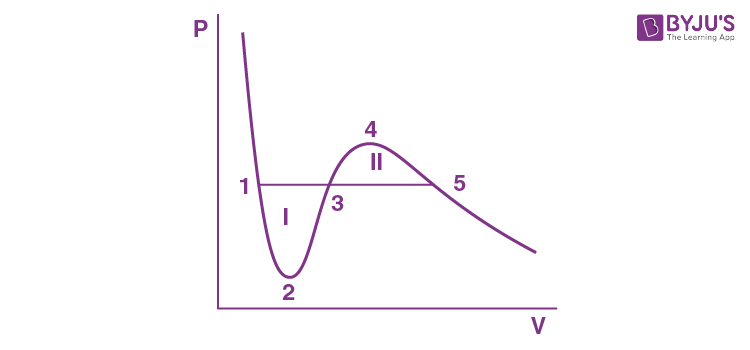Solution:

1. We apply the relation (T = constant)

This implies that the areas I and II are equal.

This reasoning is inapplicable to the cycle 1231, for example. This cycle is irreversible because it involves the irreversible transition from a single phase to a two-phase state at the point 3.